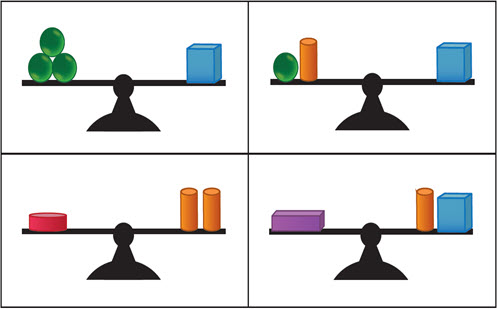Go to website

# reSolve: Algebra: Equivalence

This sequence of two lessons builds students' understanding of equivalence as balance. The equals sign is used to indicate the same value on both sides of an equation. Students develop their understanding of equivalence by looking at balancing scales with blocks of different weights. Each lesson is outlined in detail including curriculum links, vocabulary, materials needed, sample answers, discussion points and student resources. This sequence is part of the reSolve: Mathematics by Inquiry program.

Year level(s) Year 2
Audience Teacher
Purpose Teaching resource
Teaching strategies and pedagogical approaches Mathematics investigation
Keywords number patterns, mass, balance scales, mathematical expressions, number patterns, equivalence

## Curriculum alignment

Curriculum connections Numeracy
Strand and focus Number, Algebra, Build understanding, Apply understanding
Topics Properties of number, Algebraic expressions
AC: Mathematics content descriptions
ACMNA035 Describe patterns with numbers and identify missing elements
ACMNA036 Solve problems by using number sentences for addition or subtraction
National numeracy learning progression Number patterns and algebraic thinking
Multiplicative strategies - MuS3, MuS1